# GA without Phenotype

Taking the introductory example of Goldberg  in the preceding chapters we can generalize this example a bit more.We assume a world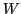consisting of events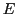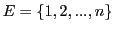. These events can be produced by a population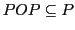(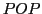in the following abbreviated as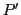) or - in simple cases - a member of the populationas such is an event.

The setrepresents the set of all possible patterns, which can be represented within a population (E.g.: If we use binary encoding of information then a population with a length of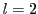would have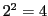many possible patterns. Thus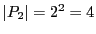.). The set of all possible combinations of information length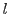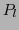is usually only a theoretical concept compared to the real population, which therefore is usually only a subset of this theoretical set(E.g.: The set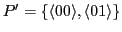is a subset of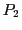.).

The fitness function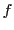maps these events into some value space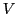like. This value spacehas to follow some ordering relation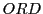with(like). Thus with the fitness functionone can compute for every member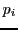of the seta fitness value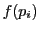. These fitness values can be ordered. Corresponding to their 'size' do these values indicate the success of an individual and summed up the success of the real population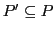as a whole.

The individuals with the least success will be suppressed and those with the highest success will be supported. This is a selection process - also often called 'recombination' - with a selection function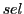mapping the old population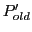in a new population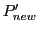.

After this selection one can apply a mating function which works like a mixing function usually called crossover. During such a crossover-mixing one takes two individualsof, takes a random position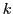in these individuals and cuts all positions fromto the end of the individual and exchanges these marked parts of each individual with each other. Thusand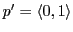with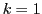would produce the 'new' members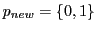and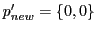. This does not change the overall fitness, and - as one can easily verify - the repetition of crossover in next cycles will not change this situation. Therefore crossover does not guarantee that a given set of informations can be mixed in a way that the whole set reaches the theoretical maximum. The crossover-set of a populationwill be written as. Then we can state the postulate, that the difference between the Maximal Fitness Set of a real populationcalledand the sum of the fitness values of a crossover-setcan not be negative: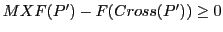.

Because the idea is to reach the maximal values of fitness for a populationone has to allow an additional operation introducing new elements by random if crossover reaches its limits. These new elements can be 'bad' or can be 'good' according to the fitness function f. The probability to get some 'good' values depends in the general case from the ratio of 'still available' good values and 'already possessing' good ones. The 'proportion' depends from the number of elements as well as of the distribution of 'good' ones to 'bad' ones. Because we assume an ordering of the values based on the fitness functionone can compute the amount of values which are 'better' incompared to the values inas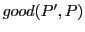. Then we have the probability to get 'better' values than given inas ProbGood=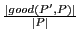. The probability to get a bad value is then. Thus if the populationbecomes 'better' then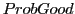decreases andincreases. From this follows that a populationwill never be 'constant' but will 'oscillate'. Thus in the case of a set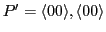compared to a setwe would have the set of 'directly available good values' inas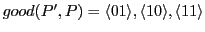with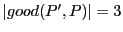and therefore ProbGood=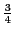.

But this general considerations have to be re-considered because the mutation operator has the constraint that he has to operate on the set of available patterns in, e.g.. Mutation can - usually - change only one position of l-many. The probability to change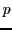to a 'better' value depends therefore from the probability of a 'positive' change in the actual pattern. In case of binary encoding only a change from '0' to '1' can increase the value. Thus the proportion of 'zeros' to 'ones'if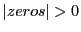, otherwise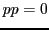. The complement of bad values is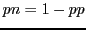. Thus a single pattern would oscillate between high probabilities for improvements and high probabilities for becoming weaker.

If we allow only one mutation in the whole population then we have a disjunction of all the available valuesIn a set of patterns which are in the beginning randomly distributed the probabilities for becoming better oder becoming more worse should be 'equal'. But because selection supports the 'better' ones the distribution will be forced to a greater 'uniformity' having more 'good' values than 'bad' ones. When such a process of increasing uniformity happens then the overall probabilities would tend to behave more and more 'monotonously'.

The 'combined effect' of selection and mutation then should be that a whole population should 'oscillate' between a 'good' and a 'bad' population.

To overcome the drawback of falling back again the population should have some protection mechanisms to inhibit mutations to become effective if the result of the mutations is weaker than the given patterns. In artificial devices built intentiously by some experts this is possible.

To sum up some of the assumed constraints:

1. Crossover needs at least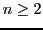positions to be applied.
2. There must be at least two individualswhich differ in at least one position, that crossover induces a change.
3. A crossover-induced-change can individually increase the value or decrease it, but the sum of both values stays the same. Therefore crossover is redundant with regard to the sum of all values in a population.
4. If it holds that for the populationthe sum of all fitness values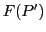is less than the maximum fitness valuesthen crossover alone can not improve the valueof a population.

Remark: This 'Generalization No.1' is - as the number '1' indicates - not the only and not the 'last' version, how one can generalize the problem of genetic algorithms. Therefore look please to further versions of these generalizations. Especially the statement the sum of both values stays the same. Therefore crossover is redundant with regard to the sum of all values in a population'' is not completely true. This will be discussed in the 'Generalizations No.2' (see 5.4).

Illustrating these ideas step by step.

Subsections
Gerd Doeben-Henisch 2013-01-14